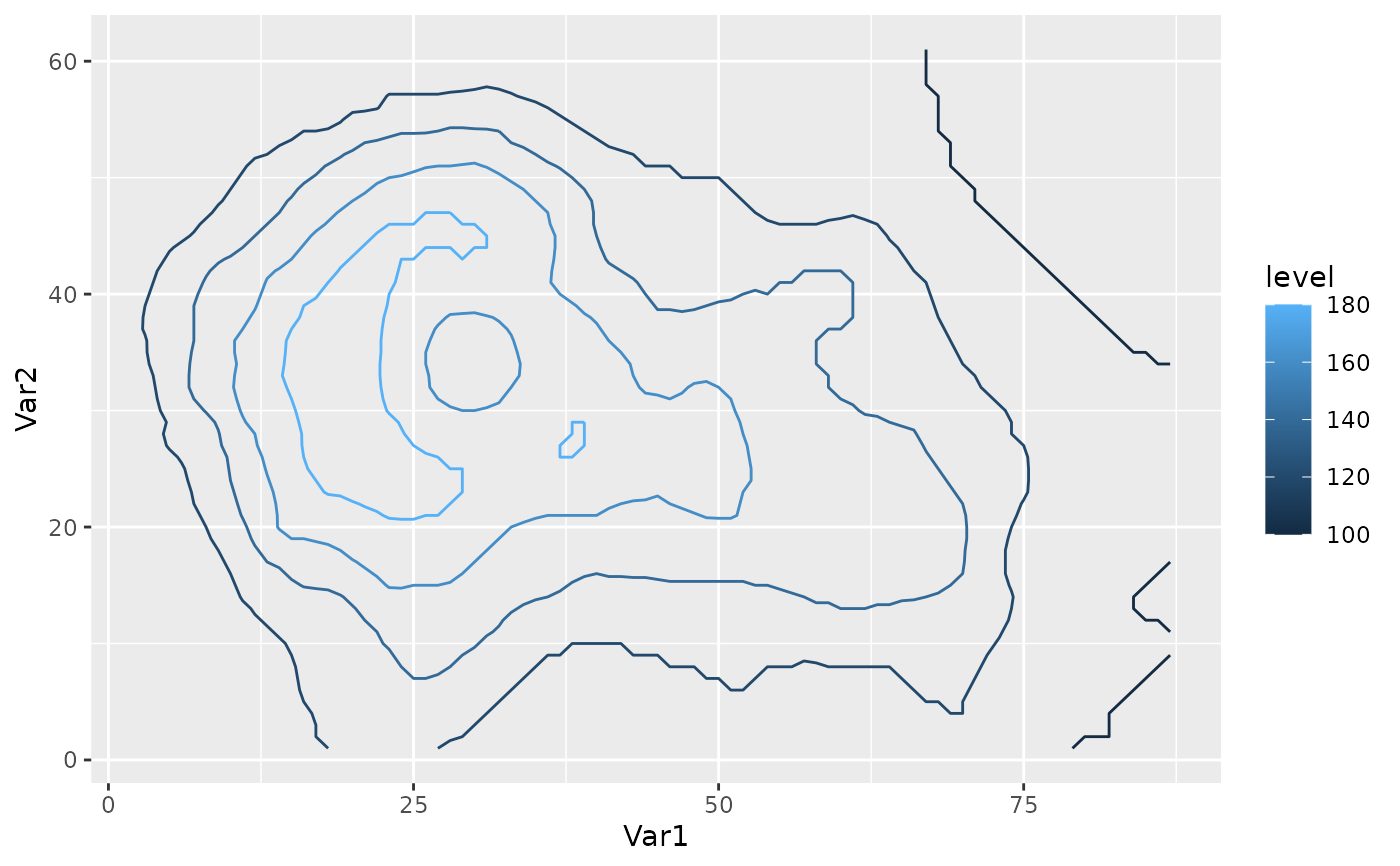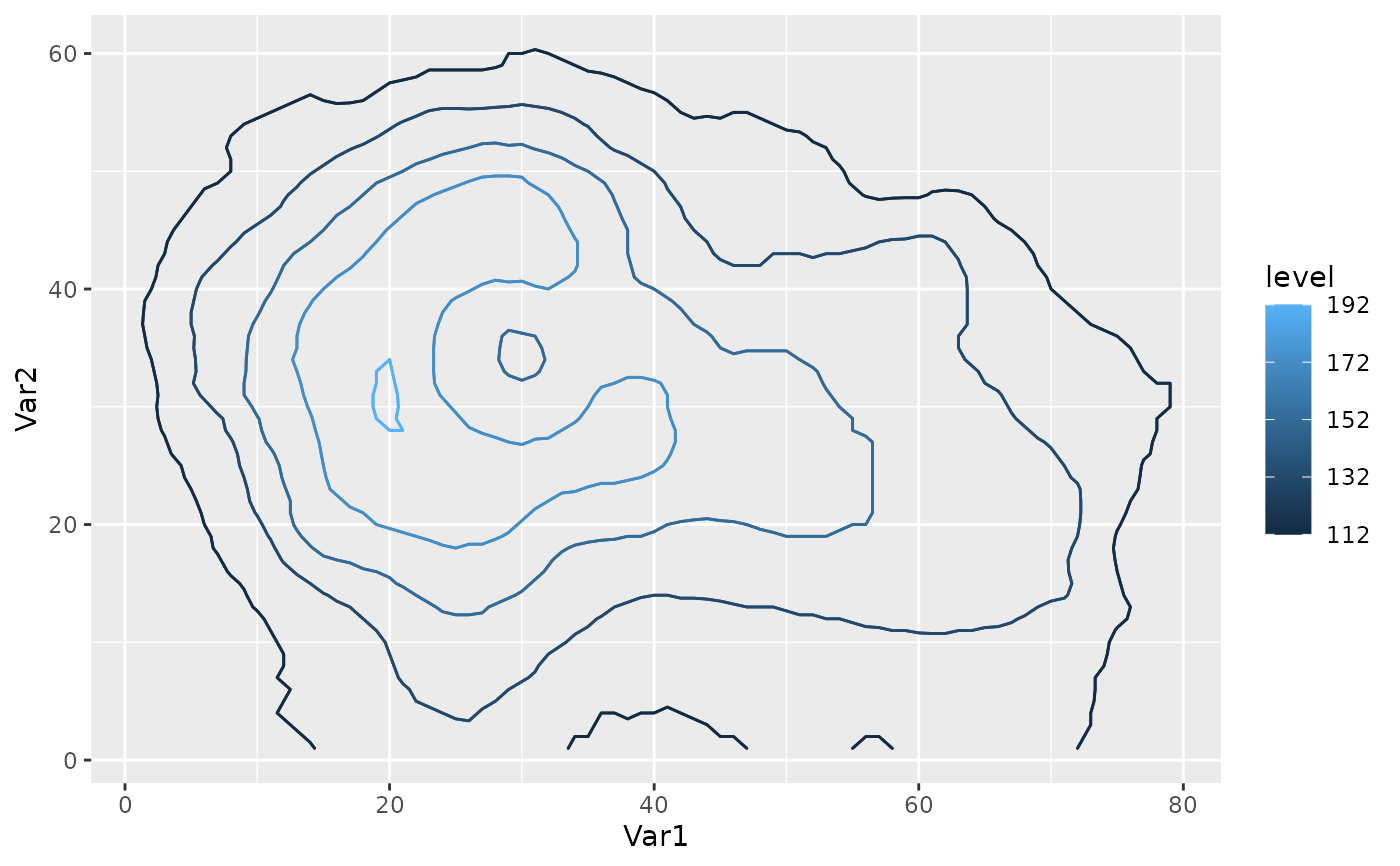Functions that return functions suitable to use as the breaks argument in ggplot2's continuous scales and in geom_contour_fill.

MakeBreaks(binwidth = NULL, bins = 10, exclude = NULL)

AnchorBreaks(anchor = 0, binwidth = NULL, exclude = NULL, bins = 10)

## Arguments

binwidth

width of breaks

bins

number of bins, used if binwidth = NULL

exclude

a vector of breaks to exclude

anchor

anchor value

## Value

A function that takes a range as argument and a binwidth as an optional argument and returns a sequence of equally spaced intervals covering the range.

## Details

MakeBreaks is essentially an export of the default way ggplot2::stat_contour makes breaks.

AnchorBreaks makes breaks starting from an anchor value and covering the range of the data according to binwidth.

Other ggplot2 helpers: DivideTimeseries(), WrapCircular(), geom_arrow(), geom_contour2(), geom_contour_fill(), geom_label_contour(), geom_relief(), geom_streamline(), guide_colourstrip(), map_labels, reverselog_trans(), scale_divergent, scale_longitude, stat_na(), stat_subset()

## Examples


my_breaks <- MakeBreaks(10)
my_breaks(c(1, 100))
#>     0  10  20  30  40  50  60  70  80  90 100
my_breaks(c(1, 100), 20)    # optional new binwidth argument ignored
#>     0  10  20  30  40  50  60  70  80  90 100

MakeBreaks()(c(1, 100), 20)  # but is not ignored if initial binwidth is NULL
#>    0  20  40  60  80 100

# One to one mapping between contours and breaks
library(ggplot2)
binwidth <- 20
ggplot(reshape2::melt(volcano), aes(Var1, Var2, z = value)) +
geom_contour(aes(color = after_stat(level)), binwidth = binwidth) +
scale_color_continuous(breaks = MakeBreaks(binwidth))#Two ways of getting the same contours. Better use the second one.
ggplot(reshape2::melt(volcano), aes(Var1, Var2, z = value)) +
geom_contour2(aes(color = after_stat(level)), breaks = AnchorBreaks(132),
binwidth = binwidth) +
geom_contour2(aes(color = after_stat(level)), breaks = AnchorBreaks(132, binwidth)) +
scale_color_continuous(breaks = AnchorBreaks(132, binwidth))